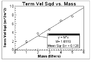# Experimental Derivation of the Drag Force

• koko122

#### koko122

I am trying to experimentally derive the drag equation. I have dropped coffee filters with varying masses and determined their resulting terminal velocity. I plotted the data and found that the mass of the coffee filter is proportional to the terminal velocity squared. I was now wondering how I can use this to derive the drag equation, which states: F_D = .5CpAv2

At terminal velocity the known constant gravitational force is equal to the unknown drag force; equate them and do a bit of algebra and you'll have the ##v^2## dependency. You will also have to do some measurements with a constant mass and different surface areas to trade out the dependency on area.

Would you be so kind to explain this a bit further. The graph I obtained is attached.

#### Attachments

•Screen Shot 2014-08-30 at 7.55.41 PM.png
5.2 KB · Views: 515
At terminal velocity the filter is not accelerating. So the forces acting on it must sum to zero. You know what the two forces are.

That's obvious but from the graph that I have provided can I derive that mg=dv^2 where d is the drag constant? Or can I show that the proportionality constant is equal to g/d somehow?

The graph shows the following:
$${v_{\textrm{T}}}^{2} \propto m$$
and from this I want to show that:
$$\Sigma F=mg-D{v_{\textrm{T}}}^{2}=0$$
So that the drag force can be defined as following:
$$F_{drag}=Dv^{2}$$

So...

mg = .5CpAv2

v2 = mg/.5CpA

The standard equation for a straight line is..

y = slope*x + constant

but constant = 0

So in this case

y = v2
x = mass m

and the slope of the graph

= g/.5CpA

You know the slope.
You know the area A
You know g

That just leaves Cp.

Sorry had to edit a few typos in this post.

That's obvious but from the graph that I have provided can I derive that mg=dv^2 where d is the drag constant? Or can I show that the proportionality constant is equal to g/d somehow?

The graph shows the following:
$${v_{\textrm{T}}}^{2} \propto m$$
and from this I want to show that:
$$\Sigma F=mg-D{v_{\textrm{T}}}^{2}=0$$
I don't see how that would follow using only the graph. It follows from Newton's second law, and that ∑F=0 at a constant velocity.

See C. Watters post #6. Cp is the only unknown in the expression for the slope, so it can be calculated.

Ya that makes sense. Would it be correct to infer from the graph that the drag force is equal to: $F_drag=Dv^2$, where D is just a general drag constant?

I don't think you can call D a "general" drag constant because D includes A (the area of the object). So it's specific to that size of filter cone rather than cones in general.

D = .5CpA

Had to edit for typo again.

I don't think you can call D a "general" drag constant because D includes A (the area of the object). So it's specific to that size of filter cone rather than cones in general.

D = .5CpA

Had to edit for typo again.
And wouldn't Cp include the density of air as well? That has to be in the drag force expression somewhere.

From http://en.wikipedia.org/wiki/Drag_(physics)#Drag_at_high_velocity
we have
$$F_{drag}=\frac{1}{2} \ \rho_{air} \ C_d \ v^2$$
where Cd is dimensionless -- and I'm pretty sure depends on the object's shape.